## LIBOCAS - Library implementing OCAS solver for training linear SVM classifiers from large-scale data

Vojtech Franc, Soeren Sonnenburg

#### Introduction

The library implements Optimized Cutting Plane Algorithm (OCAS) for training linear SVM classifiers from large-scale data. The computational effort of OCAS scales with O(m log m) where m is the sample size. In an extensive empirical evaluation OCAS significantly outperforms current state of the art SVM solvers, like SVM^light, SVM^perf and BMRM, achieving speedups of over 1,000 on some datasets over SVM^light and 20 over SVM^perf, while obtaining the same precise Support Vector solution. OCAS even in the early optimization steps shows often faster convergence than the so far in this domain prevailing approximative methods SGD and Pegasos. Effectively parallelizing OCAS we were able to train on a dataset of size 15 million examples (itself about 32GB in size) in just 671 seconds --- a competing string kernel SVM required 97,484 seconds to train on 10 million examples sub-sampled from this dataset.The library also implements COFFIN framework for efficient training of translation invariant image classifiers from virtual examples. As an example, we used the implemented framework to train a linear SVM on a gender classification dataset of almost 5 million images on a plain notebook with just 4GB of memory.

#### Features

• SVM solvers for training linear classifiers from large scale-data.
• Two-class and genuine multi-class SVM formulations.
• A stand alone application and MEX interface for Matlab.
• Optimized for features represented by different data types: sparse/dense, double/single precision, int8.
• Allows training two-class SVM classifier with additional constraints enforcing a subset of selected weights to be non-negative.
• Reads examples from SVM^light format.
• Parallelized version of the two-class SVM solver.
• Allows using different C for each training example (only for Matlab).
• Training translation invariant image classifier from virtual examples using LBP features.
• Functions for computing image features based on Local Binary Patterns (LBP).

#### Problem formulation

OCAS solver is currently implemented for training binary (two-class) and multi-class SVM classifiers:

1. Binary classification problem: OCAS solves the following unconstrained convex optimization taskwhere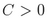is the regularization constant and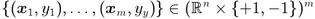are training examples. The result is the parameter vector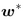of the linear rule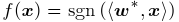which assigns vector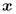a class label +1 and/or -1.

2. Multi-class classification probem: OCAS solves the following unconstrained convex optimization task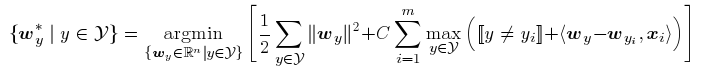whereis the regularization constant,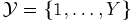is a finite set of labels and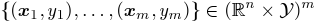are training examples. The result is a set of parameter vectors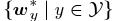of the linear rule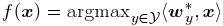which assigns vectora class label from.

GNU/Linux.